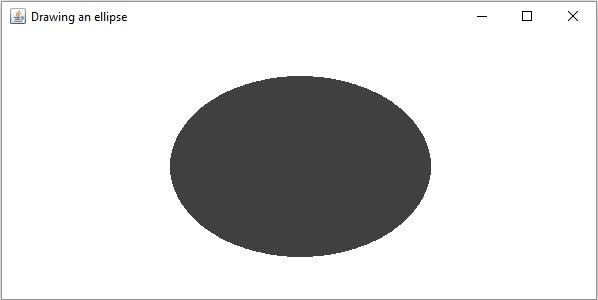# How to draw a filled ellipse in OpenCV using Java?

The org.opencv.imgproc package of Java OpenCV library contains a class named Imgproc this class provides various methods to process an input image. It provides a set of methods to draw geometrical shapes on images.

This class provides a method named ellipse() using this you can draw an ellipse on an image, one of the variants of this method allows you to specify the line type as one of the parameters including −

• A Mat object representing the image on which the ellipse is to be drawn.

• A RotatedRect object (The ellipse is drawn inscribed in this rectangle.)

• A Scalar object representing the color of the Rectangle(BGR).

If you pass Imgproc.FILLED as the parameter this method generates a filled eclipse.

## Example

import org.opencv.core.Core;
import org.opencv.core.Mat;
import org.opencv.core.Point;
import org.opencv.core.RotatedRect;
import org.opencv.core.Scalar;
import org.opencv.core.Size;
import org.opencv.highgui.HighGui;
import org.opencv.imgcodecs.Imgcodecs;
import org.opencv.imgproc.Imgproc;
public class DrawingFilledEllipse {
public static void main(String args[]) {
//Reading the source image in to a Mat object
//Drawing an ellipse
RotatedRect box = new RotatedRect(new Point(300, 200), new Size(260, 180),180);
Scalar color = new Scalar(64, 64, 64);
int thickness = Imgproc.FILLED;
Imgproc.ellipse(src, box, color, thickness);
//Saving and displaying the image
Imgcodecs.imwrite("arrowed_line.jpg", src);
HighGui.imshow("Drawing an ellipse", src);
HighGui.waitKey();
}
}

## Output

On executing, the above program generates the following window −